Expression of a variable from formula - math word problems - page 8

1. Body diagonalCuboid with base 7cm x 3,9cm and body diagonal 9cm long. Find the height of the cuboid and the length of the diagonal of the base,
2. Sugar cubesThe glass has 600 ml of tea, which represents 80% of the volume of the glass. If you put twenty regular sugar cubes of 2 cm in the tea, how many ml of tea are poured?
3. Rhombus and diagonalsThe a rhombus area is 150 cm2 and the ratio of the diagonals is 3:4. Calculate the length of its height.
4. Salary in enterprise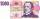The average salary in the company is 27 000 CZK, 30% of workers have the lowest average income of 19 thousand CZK. There were an increase in the salary in this group by 2%. How much % increased the average salary across the company?
5. Divide money 2Ben and Dan had the same amount of money at the start. When Ben gave 300 to Dan, the ratio of Ben 's money to Dan's money became 2:3. How much money did each have at first?
6. BookshelveBookshelve with an original price of € 200 twice become cheaper. After the second discounted by 15% the price was € 149.60. Determine how much % become cheaper for the first time.
7. Cube wallThe perimeter of one cube wall is 120 meters. Calculate the surface area and the body diagonal of this cube.
8. Isosceles trapezoidCalculate the content of an isosceles trapezoid whose bases are at ratio 5:3, the arm is 6cm long and it is 4cm high.
9. The depositDeposit has been placed for one year at an annual rate of 4.5%. After the added interst amount rose to € 2,612.5. Determine initial deposit.
10. Painters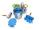Five painters painting the fence for eight days. How many days over will take work if paint the fence only four decorators?
11. On the farm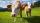On the farm were housed 70 heads of cattle with food supply for 180 days. After 60 days, 10 head of cattle sold, and for the next 30 days they sold 10 more. How many days is sufficient supply of feed for the remaining cattle?
12. TrapezoidHow long are the trapezoid bases with area 24 cm2 and height 3 cm. One base is 3 times longer than the shorter.
13. Circle and rectangleA rectangle with sides of 11.7 cm and 175 mm is described by circle. What is its length? Calculate the content area of the circle described by this circle.
14. Surface area of cylinder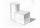Determine the lateral surface of the rotary cylinder which is circumscribed cube with edge length 5 cm.
15. Outer anglesThe outer angle of the triangle ABC at the A vertex is 71°40 ' outer angle at the vertx B is 136°50'. What size has the inner triangle angle at the vertex C?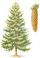On a weekly forest brigade operates 12 students. After plant one hundred spruces get x CZK, anfter one hundred pines y CZK. How many CZK got one student for one day if planted spruces 25000 and 30000 pines week?
17. Prism basesVolume perpendicular quadrilateral prism is 360 cm3. The edges of the base and height of the prism are in the ratio 5:4:2 Determine the area of the base and walls of the prism.
18. PrismCalculate the height of the prism having a surface area 448.88 dm² wherein the base is square with a side of 6.2 dm. What will be its volume in hectoliters?
19. Cuboid and eq2Calculate the volume of cuboid with square base and height 6 cm if the surface area is 48 cm2.
20. Inscribed circleXYZ is right triangle with right angle at the vertex X that has inscribed circle with a radius 5 cm. Determine area of the triangle XYZ if XZ = 14 cm.

Do you have an interesting mathematical word problem that you can't solve it? Enter it, and we can try to solve it.

To this e-mail address, we will reply solution; solved examples are also published here. Please enter e-mail correctly and check whether you don't have a full mailbox.